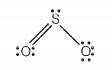Which of the following statements are correct for SO2 gas?

a. It acts as a bleaching agent in moist conditions.

b. Its molecule has linear geometry.

c. Its dilute solution is used as a disinfectant.

d. It can be prepared by the reaction of dilute H2SO4 with metal sulphide.

1. (a, b)
2. (b, c)
3. (c, d)
4. (a, c)

Hint: SO2 does not have linear geometry it has a bent shape

(a). In moist conditions SO2 gas acts as a bleaching agent. It is a correct statement
(b) The structure of SO2 is bent, not linear due to the bond pair-lone pair repulsion structure is as follows:Hence, second statement is incorrect.

(c). Its dilute solution is used as a disinfectant. The third statement is correct.

(d). It can be prepared by the reaction of O2 with sulphide ore

4 FeS2   +   11O2   →   2Fe2O3   +   8 SO2
while metal on treatment with H2SO4 produces H2S.

Hence, statement d is incorrect.

Hence, option 4th is the correct answer